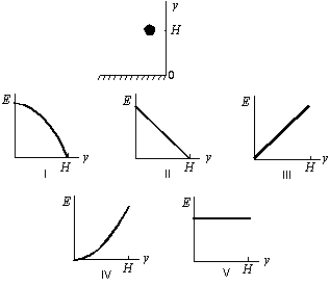# Fundamentals of Physics Study Set 2

Physics & Astronomy

## Quiz 8 : Potential Energy and Conservation of EnergyStudy FlashcardsLooking for Introductory Physics Homework Help?

## Quiz 8 :Potential Energy and Conservation of Energy

Question TypeA body at rest in a system is capable of doing work if:
Free
Multiple Choice

D

Tags
Choose question tagA golf ball is struck by a golf club and falls on a green eight feet above the tee.The potential energy of the Earth-ball system is greatest:
Free
Multiple Choice

E

Tags
Choose question tagSuppose that the fundamental dimensions are taken to be: force (F), velocity (V)and time (T).The dimensions of potential energy are then:
Free
Multiple Choice

B

Tags
Choose question tagThe sum of the kinetic and potential energies of a system of objects is conserved:
Multiple Choice
Tags
Choose question tagA ball is held at a height H above a floor.It is then released and falls to the floor.If air resistance can be ignored, which of the five graphs below correctly gives the mechanical energy E of the Earth-ball system as a function of the altitude y of the ball?Multiple Choice
Tags
Choose question tagTwo particles interact by conservative forces.In addition, an external force acts on each particle.They complete round trips, ending at the points where they started.Which of the following must have the same values at the beginning and end of this trip?
Multiple Choice
Tags
Choose question tagA force on a particle is conservative if:
Multiple Choice
Tags
Choose question tagA 6.0-kg block is released from rest 80 m above the ground.When it has fallen 60 m its kinetic energy is approximately:
Multiple Choice
Tags
Choose question tagWhich one of the following five quantities CANNOT be used as a unit of potential energy?
Multiple Choice
Tags
Choose question tagTwo objects interact with each other and with no other objects.Initially object A has a speed of 5 m/s and object B has a speed of 10 m/s.In the course of their motion they return to their initial positions.Then A has a speed of 4 m/s and B has a speed of 7 m/s.We can conclude:
Multiple Choice
Tags
Choose question tagA nonconservative force:
Multiple Choice
Tags
Choose question tagThe wound spring of a clock possesses:
Multiple Choice
Tags
Choose question tagOnly if a force on a particle is conservative:
Multiple Choice
Tags
Choose question tagAn elevator is rising at constant speed.Consider the following statements: I.the upward cable force is constant II.the kinetic energy of the elevator is constant III.the gravitational potential energy of the Earth-elevator system is constant IV.the acceleration of the elevator is zero V.the mechanical energy of the Earth-elevator system is constant
Multiple Choice
Tags
Choose question tagNo kinetic energy is possessed by:
Multiple Choice
Tags
Choose question tagA 0.20-kg particle moves along the x axis under the influence of a conservative force.The potential energy is given by U(x)= (8.0 J/m2)x2 + (2.0 J/m4)x4, Where x is in coordinate of the particle.If the particle has a speed of 5.0 m/s when it is at x = 1.0 m, its speed when it is at the origin is:
Multiple Choice
Tags
Choose question tagA force of 10 N holds an ideal spring with a 20-N/m spring constant in compression.The potential energy stored in the spring is:
Multiple Choice
Tags
Choose question tagA good example of kinetic energy is provided by:
Multiple Choice
Tags
Choose question tagA 0.50-kg block attached to an ideal spring with a spring constant of 80 N/m oscillates on a horizontal frictionless surface.The total mechanical energy is 0.12 J.The greatest extension of the spring from its equilibrium length is:
Multiple Choice